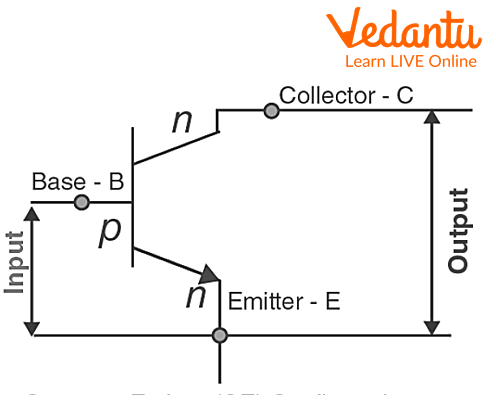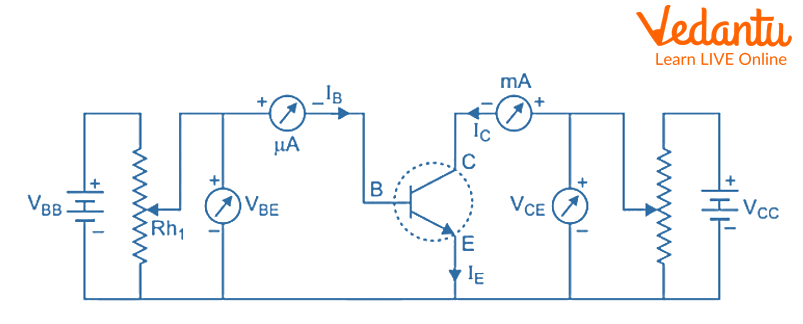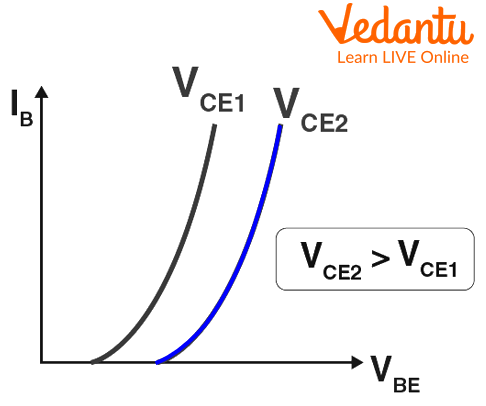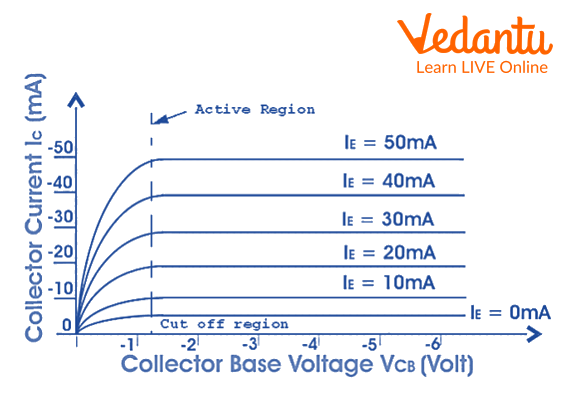Courses
Courses for Kids
Free study material
Offline Centres
More

# Common Emitter ConfigurationLast updated date: 25th Nov 2023
Total views: 57.9k
Views today: 1.57k## Common Emitter Configuration: An Introduction

Known as a common emitter layout, this arrangement places the emitter between the collector and the base. The emitter and base form the connection for the input circuit, while the collector and emitter provide the connection for the output circuit. Because the emitter is shared by the input and output circuits, the setup is known as having a common emitter. The NPN and PNP transistor's shared emitter configuration.

## Common Emitter Configuration

Since the emitter terminal is grounded in a common emitter configuration, this design is often referred to as a grounded emitter configuration. The terms common emitter configuration, common emitter amplifier, and CE amplifier are also frequently used. The most popular transistor arrangement is known as a common emitter (CE). When a significant current gain is required, common emitter amplifiers are utilised.

The base and emitter terminals are used for the input signal, while the collector and emitter terminals are used for the output signal. As a result, a transistor's emitter terminal serves as both an input and an output terminal, earning the term "common emitter configuration."Common emitter configuration

VBE stands for the supply voltage between the base and the emitter, while VCE stands for the supply voltage between the collector and the emitter. In a common emitter arrangement, input current (also known as base current or IB) and output current (also known as collector current or IC) are written as letters.

## Base Current Amplification Factor

The Base current amplification factor of common emitter configuration is given by the difference between the change in collector current (IC) and the change in base current (IB). It is indicated by $\beta$$\beta$.

$\beta =\frac{\mathrm{\Delta }{I}_{C}}{\mathrm{\Delta }{I}_{B}}$$\beta = \dfrac{{\Delta {I_C}}}{{\Delta {I_B}}}$

## Collector current and Current Gain

### Collector Current

In common emitter configuration the collector current is given by using the following equation. The equation relates the input current IB with the output current IC in the CE configuration.

${I}_{E}={I}_{C}+{I}_{B}$${I_E} = {I_C} + {I_B}$

${I}_{C}=\alpha {I}_{E}+{I}_{CBO}$${I_C} = \alpha {I_E} + {I_{CBO}}$

By using the above two equations and solving them we get:

${I}_{C}=\frac{\alpha }{1-\alpha }{I}_{B}+{I}_{CEO}$${I_C} = \dfrac{\alpha }{{1 - \alpha }}{I_B} + {I_{CEO}}$

Where,

IC - Collector current

IE- Emitter current

$\alpha$$\alpha$- Current amplification factor

IB - Base current

ICEO - When the base is open then the collector-emitter current

ICBO - When the output is open then the collector base current

### Current Gain

A current gain in common emitter configuration can be written as:

$\beta =\frac{\mathrm{\Delta }{I}_{C}}{\mathrm{\Delta }{I}_{B}}$$\beta = \dfrac{{\Delta {I_C}}}{{\Delta {I_B}}}$

Where $\beta$$\beta$ is current gain and IC change in collector current and IB change in base current. $\mathrm{\Delta }{I}_{C}$$\Delta {I_C}$ is greater than $\mathrm{\Delta }{I}_{B}$$\Delta {I_B}$. $\beta$$\beta$ is greater than 1, in common emitter configuration.

## Input and Output Characteristics

### Input Characteristics

The connection between the input current, or base current (IB), and the input voltage, or base-emitter voltage (VBE)., is described by the input characteristics of the common emitter configuration.

### Input and Output Circuit DiagramDiagram of the circuit used to analyse the input and output characteristics of a transistor's common emitter arrangement.

Draw two lines first—one vertical and one horizontal. The vertical line represents the y-axis and the x-axis by the horizontal line. The input voltage (VBE) is taken along the x-axis (horizontal line), and the input current or base current (IB), is taken along the y-axis (vertical line).Input characteristic of CE configuration

The input voltage VBE is raised from zero volts to various voltage levels while the output voltage VCE is maintained at zero volts. The matching input current (IB) for each voltage level of the input voltage (VBE) is kept track of.

### Output Characteristics

The connection between output current (IC) and output voltage (VCE) is described by the output characteristics of the common emitter configuration.

Draw two lines first—one vertical and one horizontal. The y-axis is represented by the vertical line, and the x-axis by the horizontal line. The output voltage (VCE) is taken along the x-axis, while the output current (IC), also known as the collector current, is taken along the y-axis (vertical line).The output characteristic in CE configuration

The output voltage VCE is raised from zero volts to various voltage levels to establish the output characteristics, while the input current, or base current, IB is maintained constant at 0 A. The associated output current (IC) for each level of the output voltage is kept track of.

## Interesting Facts

• Chips are intricate structures composed of layers of circuitry linking the components and millions, or even billions, of transistors and other components. Chips have gotten stronger over time by combining more microscopic components and cramming them closer together.

• Specialized manufacturing facilities known as fabs are used to create semiconductors. Cleanrooms, which are enclosed spaces with stringent controls for airborne contaminants, humidity, and temperature, are located inside these fabs.

## Solved Problems

1. Calculate the emitter current in a transistor that has $\beta$$\beta$=40, and the base current is 0.03 mA.

Sol.

Given:

IB = 0.03 mA and $\beta$$\beta$=40

We know that,

IC = $\beta$$\beta$ IB

IC = 40 x 0.03 = 1.2 mA.

We know that,

${I}_{E}={I}_{C}+{I}_{B}$${I_E} = {I_C} + {I_B}$

IE = 1.2 + 0.03 = 1.23 mA

The emitter current comes out to be 1.23 mA.

2. Find the value of $\beta$$\beta$ when $\alpha$$\alpha$is given 0.96.

Sol.

Given:

$\alpha$$\alpha$ = 0.96

We know that

$\beta =\frac{\alpha }{1-\alpha }$$\beta = \dfrac{\alpha }{{1 - \alpha }}$

$\beta =\frac{0.96}{1-0.96}$$\beta = \dfrac{{0.96}}{{1 - 0.96}}$= 24

The value of $\beta$$\beta$ is 24 after solving the question.

## Summary

We have seen how common emitter configuration works and how it amplifies the output. The other configuration is also important to get the full knowledge of this working and the relation which are set up between them. The base amplification factor is also related to the amplification factor which will help in solving the problems of physics.

## FAQs on Common Emitter Configuration

1. Why is it called a common emitter configuration?

The common-emitter amplifier, which is frequently used as a voltage amplifier, is one of the three basic single-stage bipolar-junction-transistor (BJT) amplifier topologies in electronics. We call it the common emitter configuration because the transistor's emitter is shared by the input and output circuits, the common-emitter amplifier also goes by that name. The input signal crosses the base circuit of the transistor and ground. The transistor's collector and ground receive the output signal.

2. What are CB, CE, and CC configurations?

The emitter (E), base (B), and collector (C) are the transistor's three terminals. However, we require four terminals for the circuit connections: two terminals for input and two more for output. To get around these issues, we share a single terminal for both input and output operations. Common emitter configuration, this arrangement places the emitter between the collector and base. In the CB configuration, the transistor's base terminal will be connected in series with both the input and output terminals. In the CE configuration, the transistor's collector terminal will be connected in series with both the input and output terminals.

3. What are NPN and PNP transistors?

Negative, Positive, and Negative are referred to as NPN. Positive, Negative, and Positive is referred to as PNP. sometimes referred to as sourcing A PNP. Digital signals often have an NPN or PNP relationship. Devices that can be either NPN or PNP include sensors. You must be able to match the device's signal type in order to connect to a sensor. Current moves from the emitter to the collector of an NPN transistor. When the transistor's base receives enough current, the transmitter is turned on. An NPN transistor will turn on more often the higher the current. In contrast, current moves from the collector to the emitter in a PNP transistor. When there is no current flowing across the transistor's base, the transmitter is turned on.